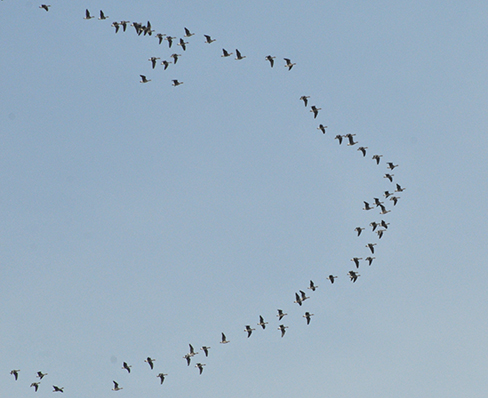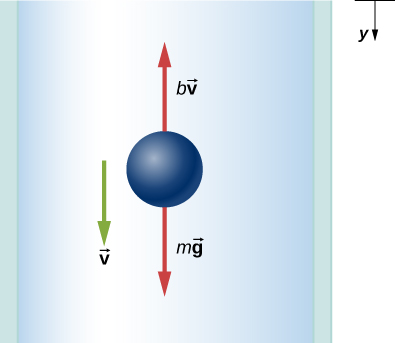# 6.4 Drag force and terminal speed  (Page 4/12)

 Page 4 / 12Geese fly in a V formation during their long migratory travels. This shape reduces drag and energy consumption for individual birds, and also allows them a better way to communicate. (credit: “Julo”/Wikimedia Commons)

In lecture demonstrations, we do measurements of the drag force on different objects. The objects are placed in a uniform airstream created by a fan. Calculate the Reynolds number and the drag coefficient.

## The calculus of velocity-dependent frictional forces

When a body slides across a surface, the frictional force on it is approximately constant and given by ${\mu }_{\text{k}}N.$ Unfortunately, the frictional force on a body moving through a liquid or a gas does not behave so simply. This drag force is generally a complicated function of the body’s velocity. However, for a body moving in a straight line at moderate speeds through a liquid such as water, the frictional force can often be approximated by

${f}_{R}=\text{−}bv,$

where b is a constant whose value depends on the dimensions and shape of the body and the properties of the liquid, and v is the velocity of the body. Two situations for which the frictional force can be represented this equation are a motorboat moving through water and a small object falling slowly through a liquid.

Let’s consider the object falling through a liquid. The free-body diagram of this object with the positive direction downward is shown in [link] . Newton’s second law in the vertical direction gives the differential equation

$mg-bv=m\frac{dv}{dt},$

where we have written the acceleration as $dv\text{/}dt.$ As v increases, the frictional force – bv increases until it matches mg . At this point, there is no acceleration and the velocity remains constant at the terminal velocity ${v}_{\text{T}}.$ From the previous equation,

$mg-b{v}_{\text{T}}=0,$

so

${v}_{\text{T}}=\frac{mg}{b}.$Free-body diagram of an object falling through a resistive medium.

We can find the object’s velocity by integrating the differential equation for v . First, we rearrange terms in this equation to obtain

$\frac{dv}{g-\left(b\text{/}m\right)v}=dt.$

Assuming that $v=0\phantom{\rule{0.2em}{0ex}}\text{at}\phantom{\rule{0.2em}{0ex}}t=0,$ integration of this equation yields

${\int }_{0}^{v}\frac{d{v}^{\prime }}{g-\left(b\text{/}m\right){v}^{\prime }}={\int }_{0}^{t}d{t}^{\prime },$

or

${-\frac{m}{b}\text{ln}\left(g-\frac{b}{m}{v}^{\prime }\right)|}_{0}^{v}={{t}^{\prime }|}_{0}^{t},$

where $v\text{'}\phantom{\rule{0.2em}{0ex}}\text{and}\phantom{\rule{0.2em}{0ex}}t\text{'}$ are dummy variables of integration. With the limits given, we find

$-\frac{m}{b}\left[\text{ln}\left(g-\frac{b}{m}v\right)-\text{ln}g\right]=t.$

Since $\text{ln}A-\text{ln}B=\text{ln}\left(A\text{/}B\right),$ and $\text{ln}\left(A\text{/}B\right)=x\phantom{\rule{0.2em}{0ex}}\text{implies}\phantom{\rule{0.2em}{0ex}}{e}^{x}=A\text{/}B,$ we obtain

$\frac{g-\left(bv\text{/}m\right)}{g}={e}^{\text{−}bt\text{/}m},$

and

$v=\frac{mg}{b}\left(1-{e}^{\text{−}bt\text{/}m}\right).$

Notice that as $t\to \infty ,v\to mg\text{/}b={v}_{\text{T}},$ which is the terminal velocity.

The position at any time may be found by integrating the equation for v . With $v=dy\text{/}dt,$

$dy=\frac{mg}{b}\left(1-{e}^{\text{−}bt\text{/}m}\right)dt.$

Assuming $y=0\phantom{\rule{0.2em}{0ex}}\text{when}\phantom{\rule{0.2em}{0ex}}t=0,$

${\int }_{0}^{y}d{y}^{\prime }=\frac{mg}{b}{\int }_{0}^{t}\left(1-{e}^{\text{−}bt\text{'}\text{/}m}\right)d{t}^{\prime },$

which integrates to

$y=\frac{mg}{b}t+\frac{{m}^{2}g}{{b}^{2}}\left({e}^{\text{−}bt\text{/}m}-1\right).$

## Effect of the resistive force on a motorboat

A motorboat is moving across a lake at a speed ${v}_{0}$ when its motor suddenly freezes up and stops. The boat then slows down under the frictional force ${f}_{R}=\text{−}bv.$ (a) What are the velocity and position of the boat as functions of time? (b) If the boat slows down from 4.0 to 1.0 m/s in 10 s, how far does it travel before stopping?

## Solution

1. With the motor stopped, the only horizontal force on the boat is ${f}_{R}=\text{−}bv,$ so from Newton’s second law,
$m\frac{dv}{dt}=\text{−}bv,$

which we can write as
$\frac{dv}{v}=-\frac{b}{m}dt.$

Integrating this equation between the time zero when the velocity is ${v}_{0}$ and the time t when the velocity is $v$ , we have
${\int }_{0}^{v}\frac{d{v}^{\prime }}{{v}^{\prime }}=-\frac{b}{m}{\int }_{0}^{t}d{t}^{\prime }.$

Thus,
$\text{ln}\frac{v}{{v}_{0}}=-\frac{b}{m}t,$

which, since $\text{ln}A=x\phantom{\rule{0.2em}{0ex}}\text{implies}\phantom{\rule{0.2em}{0ex}}{e}^{x}=A,$ we can write this as
$v={v}_{0}{e}^{\text{−}bt\text{/}m}.$

Now from the definition of velocity,
$\frac{dx}{dt}={v}_{0}{e}^{\text{−}bt\text{/}m},$

so we have
$dx={v}_{0}{e}^{\text{−}bt\text{/}m}dt.$

With the initial position zero, we have
${\int }_{0}^{x}dx\text{'}={v}_{0}{\int }_{0}^{t}{e}^{\text{−}bt\text{'}\text{/}m}dt\text{'},$

and
${x=-\frac{m{v}_{0}}{b}{e}^{\text{−}bt\text{'}\text{/}m}|}_{0}^{t}=\frac{m{v}_{0}}{b}\left(1-{e}^{\text{−}bt\text{/}m}\right).$

As time increases, ${e}^{\text{−}bt\text{/}m}\to 0,$ and the position of the boat approaches a limiting value
${x}_{\text{max}}=\frac{m{v}_{0}}{b}.$

Although this tells us that the boat takes an infinite amount of time to reach ${x}_{\text{max}},$ the boat effectively stops after a reasonable time. For example, at $t=10m\text{/}b,$ we have
$v={v}_{0}{e}^{-10}\simeq 4.5\phantom{\rule{0.2em}{0ex}}×\phantom{\rule{0.2em}{0ex}}{10}^{-5}{v}_{0},$

whereas we also have
$x={x}_{\text{max}}\left(1-{e}^{-10}\right)\simeq 0.99995{x}_{\text{max}}.$

Therefore, the boat’s velocity and position have essentially reached their final values.
2. With ${v}_{0}=4.0\phantom{\rule{0.2em}{0ex}}\text{m/s}$ and $v=1.0\phantom{\rule{0.2em}{0ex}}\text{m/s,}$ we have $1.0\phantom{\rule{0.2em}{0ex}}\text{m/s}=\left(4.0\phantom{\rule{0.2em}{0ex}}\text{m/s}\right){e}^{\text{−}\left(b\text{/}m\right)\left(10\phantom{\rule{0.2em}{0ex}}\text{s}\right)},$ so
$\text{ln}\phantom{\rule{0.2em}{0ex}}0.25=\text{−}\text{ln}\phantom{\rule{0.2em}{0ex}}4.0=-\frac{b}{m}\left(10\phantom{\rule{0.2em}{0ex}}\text{s}\right),$

and
$\frac{b}{m}=\frac{1}{10}\text{ln}\phantom{\rule{0.2em}{0ex}}4.0\phantom{\rule{0.2em}{0ex}}{\text{s}}^{\text{-1}}=0.14\phantom{\rule{0.2em}{0ex}}{\text{s}}^{\text{-1}}\text{.}$

Now the boat’s limiting position is
${x}_{\text{max}}=\frac{m{v}_{0}}{b}=\frac{4.0\phantom{\rule{0.2em}{0ex}}\text{m/s}}{0.14\phantom{\rule{0.2em}{0ex}}{\text{s}}^{\text{−1}}}=29\phantom{\rule{0.2em}{0ex}}\text{m}\text{.}$

#### Questions & Answers

a particle projected from origin moving on x-y plane passes through P & Q having consituents (9,7) , (18,4) respectively.find eq. of trajectry.
rahul Reply
definition of inertia
philip Reply
the reluctance of a body to start moving when it is at rest and to stop moving when it is in motion
charles
An inherent property by virtue of which the body remains in its pure state or initial state
Kushal
why current is not a vector quantity , whereas it have magnitude as well as direction.
Aniket Reply
why
daniel
the flow of current is not current
fitzgerald
bcoz it doesn't satisfy the algabric laws of vectors
Shiekh
The Electric current can be defined as the dot product of the current density and the differential cross-sectional area vector : ... So the electric current is a scalar quantity . Scalars are related to tensors by the fact that a scalar is a tensor of order or rank zero .
Kushal
what is binomial theorem
Tollum Reply
hello are you ready to ask aquestion?
Saadaq Reply
what is binary operations
Tollum
What is the formula to calculat parallel forces that acts in opposite direction?
Martan Reply
position, velocity and acceleration of vector
Manuel Reply
hi
peter
hi
daniel
hi
Vedisha
*a plane flies with a velocity of 1000km/hr in a direction North60degree east.find it effective velocity in the easterly and northerly direction.*
imam
hello
Lydia
hello Lydia.
Sackson
What is momentum
isijola
hello
Saadaq
A rail way truck of mass 2400kg is hung onto a stationary trunk on a level track and collides with it at 4.7m|s. After collision the two trunk move together with a common speed of 1.2m|s. Calculate the mass of the stationary trunk
Ekuri Reply
I need the solving for this question
philip
is the eye the same like the camera
EDWIN Reply
I can't understand
Suraia
same here please
Josh
I think the question is that ,,, the working principal of eye and camera same or not?
Sardar
yes i think is same as the camera
muhammad
what are the dimensions of surface tension
samsfavor
why is the "_" sign used for a wave to the right instead of to the left?
MUNGWA Reply
why classical mechanics is necessary for graduate students?
khyam Reply
classical mechanics?
Victor
principle of superposition?
Naveen Reply
principle of superposition allows us to find the electric field on a charge by finding the x and y components
Kidus
Two Masses,m and 2m,approach each along a path at right angles to each other .After collision,they stick together and move off at 2m/s at angle 37° to the original direction of the mass m. What where the initial speeds of the two particles
MB
2m & m initial velocity 1.8m/s & 4.8m/s respectively,apply conservation of linear momentum in two perpendicular directions.
Shubhrant
A body on circular orbit makes an angular displacement given by teta(t)=2(t)+5(t)+5.if time t is in seconds calculate the angular velocity at t=2s
MB
2+5+0=7sec differentiate above equation w.r.t time, as angular velocity is rate of change of angular displacement.
Shubhrant
Ok i got a question I'm not asking how gravity works. I would like to know why gravity works. like why is gravity the way it is. What is the true nature of gravity?
Daniel Reply
gravity pulls towards a mass...like every object is pulled towards earth
Ashok
An automobile traveling with an initial velocity of 25m/s is accelerated to 35m/s in 6s,the wheel of the automobile is 80cm in diameter. find * The angular acceleration
Goodness Reply
(10/6) ÷0.4=4.167 per sec
Shubhrant
what is the formula for pressure?
Goodness Reply
force/area
Kidus
force is newtom
Kidus
and area is meter squared
Kidus
so in SI units pressure is N/m^2
Kidus
In customary United States units pressure is lb/in^2. pound per square inch
Kidus

### Read also:

#### Get the best University physics vol... course in your pocket!

Source:  OpenStax, University physics volume 1. OpenStax CNX. Sep 19, 2016 Download for free at http://cnx.org/content/col12031/1.5
Google Play and the Google Play logo are trademarks of Google Inc.

Notification Switch

Would you like to follow the 'University physics volume 1' conversation and receive update notifications?ByBy Naveen TomarByBy